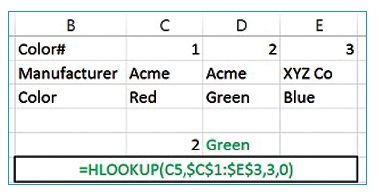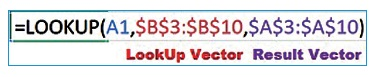# LookUp-palooza: Make the function sing in Excel

by on
in

Let’s talk lookups. Many Excel users have at least heard of the VLookUp function. Fewer have heard about HLookUp, and LookUp. Here’s an over­­view of what each of these functions does and how they’re used in the wild.

### VLookUp

If you’ve ever looked down the menu at a restaurant and then looked over to the right for the price of an item, you have performed a vertical lookup. That is what a VLookUp does. You’ve even done it in Excel when you scroll down a list looking for a particular customer, tabbed to the right to select that customer’s name and copied and pasted it to another worksheet. If you tell Excel what you want to look up, where you want to look for it, which column has the information you need and what to do if it can’t find it, it will perform the function for you.In the example, you’ll see listed a color table with a color number in column A and color name in column B. In cell D1, we’ve typed the color number for which we want to learn the color name.  In E1, we input our VLookUp. The function’s structure is basically: What, Where, Which and What If.

• “What” value is the color number we typed in C1
• “Where” is range A1:B8, with the lookup value in the first column of the table.
• “Which” is the second column or column 2.
• “What if ...” is 0 or False, meaning “Tell me you can’t find it by putting #N/A in the cell as the result. When you look up values within a range, use 1 or blank (True), which means get close without going over. If our formula had a 1 instead of 0 in the last argument, a value of 3.9 in D1 would yield Blue.

Tip: Use absolute cell references (\$A\$1:\$B\$8) to anchor your lookup table (“where”). Otherwise, as you copy it down, it will change relative to where it’s copied, so A2:B9, then A3:B10, etc. You may also use a named range.  Also, if you look up a value within a range, (1 or blank in the “what if” field) be sure to sort your lookup column in ascending order.

### HLookUpIf the V in VLookUp stands for vertical, you would be correct in guessing that the H in HLookUp stands for horizontal. In an HLookUp, we would find the lookup value across a row, and then tell Excel how many rows to look down to find the value we want returned. Like a VLookUp table needs to have the lookup value in the first column, an HLookUp table needs to have the lookup value in the top row.

### LookUpWhen the lookup value is not in the first row (HLookUp) or the first column (VLookUp), a simple LookUp function might overcome that. After specifying the lookup value, select the cell range, row or column, where you want Excel to find it. This is called the lookup vector. Then, select the corresponding range where the result value resides. This is called the result vector.